## Year 3 mental arithmetic: sets 71 and 72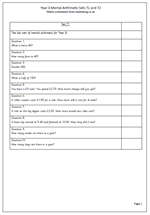Here we have the last sets of mental arithmetic questions for year 3. The complete pack of 72 sets of ten questions can be used in 12 week blocks over the three terms, using two sets each week. Probably the most important part of this whole process is to ask how children go about answering the questions and discussing with them different approaches, some of which are much quicker and easier than others.

If children can answer these last two sets of questions quickly and correctly then they will be very well set up for the next year and will have a sound base on which to progress.

Questions this week include:

doubling 2-digit and 3-digit numbers

finding halves of 3-digit multiples of 10

change from £5.00

further money problems

time questions such as the number of weeks in a year.

Year 3 mental arithmetic: sets 71 and 72

## Year 3 Mental Arithmetic: sets 69 and 70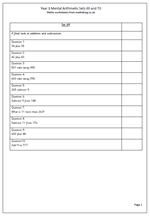A final look at addition and subtraction with these two sets of ten mental arithmetic questions for year 3. By the end of the year children should really be developing their mental arithmetic strategies, which are almost like ‘tricks of the trade’. Let’s take a look at a few of them which crop up in these questions.

1. 34 add 35 can be done in several ways such as adding the tens then adding the units and adding the two totals. Another way is to double one of the numbers and adjust by one e.g. double 34 is 68; add one to make 69.

2.  603 – 590. My favourite way to do this is adding on: add 10 to make 600 and then add 3 more to make 603. Answer is 13. This is much easier than trying to subtract 590 from 603.

3. subtract 9 from 265. This is one they should be very familiar with; take ten and add one. Of course some children will count down 9 using their fingers, but this will be much slower.

Year 3 mental arithmetic: sets 69 and 70

## Year 3 Mental Arithmetic: Sets 67 and 68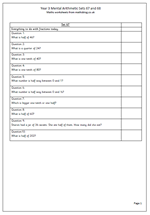We will be shortly be drawing to a close the weekly year 3 mental arithmetic. Meanwhile here is a set of questions concentrating on fractions. Always a tricky subject, often disliked by children and adults, they can, in fact, be quite good fun.

By the end of year 3 children should know that dividing by 2 will give half of a number; dividing by 4 will give a quarter of a number and dividing by 10 will give a tenth of a number.

One of the puzzling things about fractions can be that as a number gets bigger, the fraction gets smaller. So 1/10 of a number is a smaller amount than 1/4 or 1/6 of that number.

Something else which many children find tricky is finding the number half way between two other numbers, such as finding the number half way between 8 and a half and nine. Plenty of practice counting in halves and quarters will help with this.

Year 3 mental arithmetic: sets 67 and 68

## Year 3 mental arithmetic: sets 63 and 64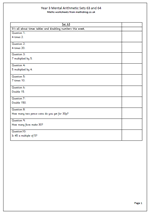We continue into the second part of the summer term with our next set of year 3 mental arithmetic questions. This week’s questions are particularly suitable for reading out loud, rather than printing and looking at, as they are all short. They concentrate on times tables, doubling and division.

The questions on times tables concentrate on the two, times, five times and ten times tables which children should know ‘off by heart’ by this stage. Question 2 is trickier as it needs to make use of the 2 times table to multiply by 20. The doubling questions look at doubling multiples of 5.

Question ten uses the term multiple which children should have come across this year, probably in terms of the answers to times tables i.e. the product of two whole numbers.

All the mental arithmetic sets of questions can be found in the Year 2 Calculating category.

Year 3 mental arithmetic_(sets 63 and 64)

## Year 3 Mental Arithmetic: Sets 61 and 62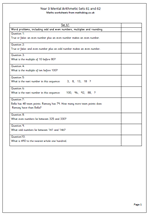This weeks’ mental arithmetic for year 3 has a variety of questions ranging from odd and even numbers to number sequences.

The Primary Framework for Mathematics lays great emphasis on odd and even numbers and it is very useful to know the pattern of adding and subtracting odd and even numbers, especially when carrying out quick checks as to whether answers are correct or not.

Children should know that:

an even number plus an even number will give and even number

an even number plus an odd number will give an odd number

an odd number plus an odd number will give an even number.

The same pattern occurs with subtraction.

The term ‘multiple’ is also expected to be understood in year 3 and in this early stage it can be thought of as:

‘a number produced by multiplying a starting whole number by another whole number’. The answers to times tables are excellent examples of multiples.

Year 3 mental arithmetic: Sets 61 and 62

## Year 3 mental artihmetic: sets 59 and 60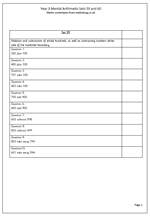As we move towards the end of the year 3 term the mental arithmetic questions become slightly harder. These two pages of addition and subtraction questions include some quite tricky problems. The first few questions are quite straightforward with adding or subtracting multiples of 100 from 3-digit numbers, although there are many children who are not at all comfortable with larger numbers. This can often be shown by asking them to count on in whole hundreds from a given number such as 34.

The last four questions look at subtraction either side of a multiple of 100. For example, at first glance 603 subtract 598 looks as if it should be done using paper and pencil methods, but it is quite easy to do by counting on.

Count on 2 from 598 to make 600. Then count on another 3 to make 603 which gives an answer of 5. No harm in using fingers to do this sort of question, especially if the question is given orally and the numbers cannot be seen.

Year 3 mental arithmetic_(sets 59 and 60)

## Year 3 Mental Arithmetic: Sets 57 and 58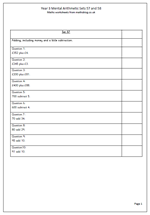We will soon be coming to the end of our sets of mental arithmetic questions for year 3, with just three more sets after these. Today the emphasis is on addition skills as well as a little subtraction.

The questions include:

adding a single digit to a 3-digit number in the context of money: e.g. £352 plus £4

adding a 2-digit number to a 3-digit multiple of 100: e.g. £400 + £88

adding a 2-digit multiple of 10 to a 2-digit number: e.g. to add 34

subtracting a single digit from a multiple of 100: e.g. 600 subtract 4

These are all important steps in becoming confident with working with numbers ‘in your head’ and they should be practiced as often as possible.

Year 3 mental arithmetic (sets 57 and 58)

## Year 3 mental arithmetic: sets 55 and 56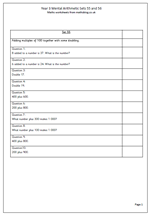Two more sets of ten questions which add to the great collection now available for year 3 children. This week there are some tricky questions which must be listened to carefully if the correct answer is to be worked out.

For example: ‘8 added to a number is 37. What is the number?’

The danger here is to add 8 to 37 rather than subtract it!

other questions cover doubling numbers in the teens. This is quite hard for year 3 children but it is hoped that they learn the answers to these off by heart as soon as possible. The rest of the set are mainly concerned with the addition of multiples of 100.

Year 3_mental arithmetic:_(sets 55 and 56)

## Year 3 mental arithmetic: sets 53 and 54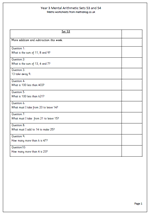I continue on with the weekly sets of mental arithmetic for Year 3. This week the questions concentrate on addition and subtraction, including adding three numbers ‘in your head’. Both the first two questions are designed so that pairs that make 10 can be spotted e.g. 11 + 8 + 9, where the easiest way to do this is to firstly add the 11 and 9 to make 20.

Children need to listen carefully to the questions to make sure that they carry out the right calculation. For example;

‘What must I add to 14 to make 25?’

Some children will here add, 14 and 25 and immediately add 14 and 25 to make 39, when they should, of course, subtract.

In a similar ay, the question:

‘How many more than 4 is 23?’

will be interpreted by some as adding 4 to 23 rather than counting on from 4 to 23.

Year 3 mental arithmetic sets 53 and 54

## Year 3 Mental Arithmetic: sets 51 and 52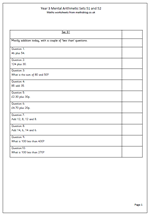This week’s year 3 mental arithmetic questions concentrate on addition, with a couple of ‘less than’ questions. Children should be getting more familiar with the various techniques they can use to answer questions ‘in their heads’.

For example:

a. it helps to look for pairs of numbers that make 10 such as 5 and 5 or 6 and 4. These pairs all need to be learnt off by heart.

b. when adding pairs of multiples of ten, use knowledge of adding single digits. If the answer to 6 + 7 is known off by heart it makes it easy to add 60 and 70.

c. when adding pounds and pence make sure the answer is using the correct units e.g. £2.70 + 20p = £2.90. (Not £2.90p)

d. when adding three or four numbers to remember that addition can be done in any order and it is often best to start with the largest number.

Year 3 mental arithmetic (sets 51 and 52)